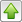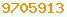# round : Java Glossary

round
To take a float or double and want the corresponding integer (possibly as a float, double, int or long) there are five basic algorithms you might use: round, ceil, floor, truncate and cover. In elementary school you learned that rounding always involves 0.5. Java and the IEEE (Institute of Electrical & Electronics Engineers) use the term in a more general sense to describe any way a number is converted from floating point to integer.

DecimalFormat automatically rounds output to the displayed number of decimal places, so there is nothing to do if you are rounding simply to make output look pretty.

• ## Integer Division

If you want to use pure integer arithmetic likely you can use one of these three forms of division.
```// integer division
// What percentage is "after" of "before" expressed as number 0..100 percent
int percent = ( after * 100 ) / before;  // truncated down
int percent = ( after * 100 + before - 1) / before;  // rounded up
int percent = ( after * 100 + before/2) / before;  // rounded```
• ## Round

Numbers less that x.5 are rounded down toward zero, and those x.5 and above are rounded up to the next integer. Unfortunately rint does not give you an easy way to round to a given number of decimal places. You have to do it by multiplying and dividing.
rint does not round 0.5 up, it rounds it up or down, whichever of the lower or higher integer is even!
```long Math.round( double );
int Math.round( float );
double Math.rint( double );

// rounding to two decimal places
double roundedValue = Math.rint( value * 100.0d ) / 100.0d;```
value round
-1.6 -2
-1.5 -2
-1.4 -1
+1.4 +1
+1.5 +2
+1.6 +2
• ## Ceiling

Numbers
`double Math.ceil( double );`
See the description of covered quotient division.
value ceil
-1.6 -1
-1.5 -1
-1.4 -1
+1.4 +2
+1.5 +2
+1.6 +2
• ## Floor

Numbers
`double Math.floor( double );`
See floored division.
value floor
-1.6 -2
-1.5 -2
-1.4 -2
+1.4 +1
+1.5 +1
+1.6 +1
• ## Truncate

Numbers
`int i = (int)d;`
value truncate
-1.6 -1
-1.5 -1
-1.4 -1
+1.4 +1
+1.5 +1
+1.6 +1
• ## Cover

Numbers are always rounded up away from zero. There is no simple function to do this.
`d < 0 ? Math.floor( d ) : Math.ceil( d );`
value cover
-1.6 -2
-1.5 -2
-1.4 -2
+1.4 +2
+1.5 +2
+1.6 +2

## Rounding to a Multiple

```// rounding m up to next highest multiple of n
int ceil = ( m + n - 1 ) / n * n;

// rounding m down to multiple of n
int floor = m / n * n;

// rounding m to nearest multiple of n
int near = ( m + n/2 ) / n * n;```

## Rounding To The Nickel

On 2013-02-04 Canada discontinued the penny. Transactions are still computed to the penny, but are rounded to the nickel. The government has suggested (but not mandated) the following rounding rules.

• Final amounts ending in .01 or .02 will be rounded down to nearest .00
• Final amounts ending in .03 or .04 will be rounded up to nearest .05
• Final amounts ending in .06 or .07 will be rounded down to nearest .05
• Final amounts ending in .08 or .09 will be rounded up to nearest .10

The formula to compute the rounded amount could be described in words as.

3. divide by 5, discarding the fraction.
4. multiply by 5

You can calculate the rounded amount in a spreadsheet with =ROUNDDOWN((A1+2)/5)*5

The Canadian Sales Tax Calculator will compute the final sale amount including sales tax both penny and nickel-rounded.

## Learning More

Oracle’s Javadoc on Math.ceil : available:
Oracle’s Javadoc on Math.floor : available:
Oracle’s Javadoc on Math.rint : available:
Oracle’s Javadoc on Math.round : available:This page is postedon the web at: http://mindprod.com/jgloss/round.html Optional Replicator mirror of mindprod.com on local hard disk J: J:\mindprod\jgloss\round.htmlPlease read the feedback from other visitors, or send your own feedback about the site.Contact Roedy. Please feel free to link to this page without explicit permission. Canadian Mind Products IP:[65.110.21.43] Your face IP:[3.239.59.31] Feedback You are visitor number